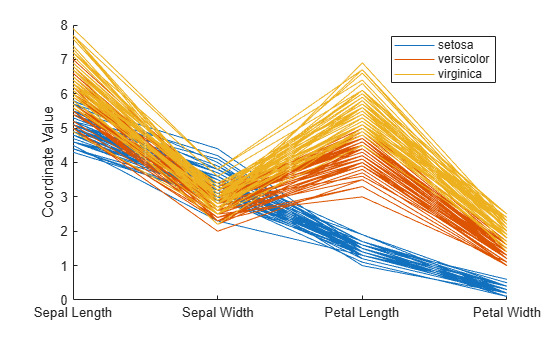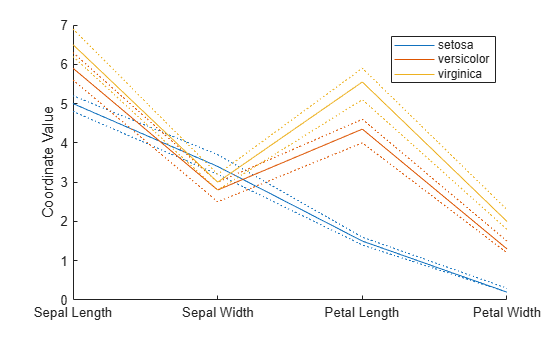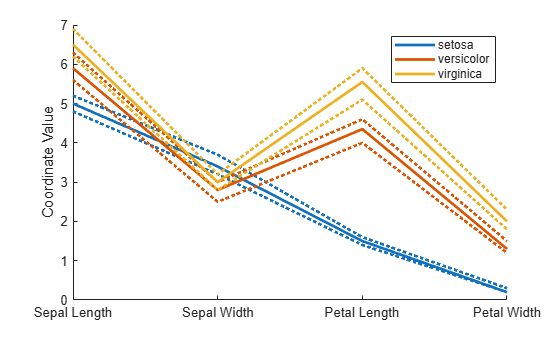Documentation

parallelcoords

Parallel coordinates plot

Description

parallelcoords(x) creates a parallel coordinates plot of the multivariate data in the matrix x. Use a parallel coordinates plot to visualize high dimensional data, where each observation is represented by the sequence of its coordinate values plotted against their coordinate indices.

example

parallelcoords(x,Name,Value) creates a parallel coordinates plot with additional options specified by one or more Name,Value pair arguments. For example, you can standardize the data in x or label the coordinate tick marks along the horizontal axis of the plot.

parallelcoords(ax,___) creates a parallel coordinates plot using the axes specified by the axes graphic object ax, using any of the previous syntaxes.

example

h = parallelcoords(___) returns a column vector of handles to the Line objects created by parallelcoords, with one handle for each row of x.

Examples

collapse all

Load the Fisher iris sample data.

The data contains four measurements (sepal length, sepal width, petal length, and petal width) from three species of iris flowers. The matrix meas contains all four measurements for each of 150 flowers. The cell array species contains the species name for each of the 150 flowers.

Create a cell array that contains the name of each measurement variable in the sample data.

labels = {'Sepal Length','Sepal Width','Petal Length','Petal Width'};

Create a parallel coordinate plot using the measurement data in meas. Use a different color for each group as identified in species, and label the horizontal axis using the variable names.

parallelcoords(meas,'Group',species,'Labels',labels)The resulting plot contains one line for each observation (flower). The color of each line indicates the flower species.

Load the Fisher iris sample data.

The data contains four measurements (sepal length, sepal width, petal length, and petal width) from three species of iris flowers. The matrix meas contains all four measurements for each of 150 flowers. The cell array species contains the species name for each of the 150 flowers.

Create a cell array that contains the name of each measurement variable in the sample data.

labels = {'Sepal Length','Sepal Width','Petal Length','Petal Width'};

Create a parallel coordinates plot using the measurement data in meas. Plot only the median, 25 percent, and 75 percent quartile values for each group identified in species. Label the horizontal axis using the variable names.

parallelcoords(meas,'group',species,'labels',labels,...
'quantile',.25)The plot shows the median values for each group as a solid line and the quartile values as dotted lines of the same color. For example, the solid blue line shows the median value measured for each variable on setosa irises. The dotted blue line below the solid blue line shows the 25th percentile of measurements for each variable on setosa irises. The dotted blue line above the solid blue line shows the 75th percentile of measurements for each variable on setosa irises.

Load the Fisher iris sample data.

The data contains four measurements (sepal length, sepal width, petal length, and petal width) from three species of iris flowers. The matrix meas contains all four measurements for each of 150 flowers. The cell array species contains the species name for each of the 150 flowers.

Create a cell array that contains the name of each measurement variable in the sample data.

labels = {'Sepal Length','Sepal Width','Petal Length','Petal Width'};

Create a parallel coordinates plot using the measurement data in meas. Plot only the median, 25 percent, and 75 percent quartile values for each group identified in species. Label the horizontal axis using the variable names. Set the line width to 2.

parallelcoords(meas,'group',species,'labels',labels,...
'quantile',.25,'LineWidth',2)Specifying 'LineWidth' in this way sets the width of every line in the plot to 2.

Recreate the parallel coordinates plot, but this time, use handles to increase the width of only the line representing the median value for each measurement made on irises in the setosa group.

h = parallelcoords(meas,'group',species,'labels',labels,...
'quantile',.25)h =
9x1 Line array:

Line    (median)
Line    (lower quantile)
Line    (upper quantile)
Line    (median)
Line    (lower quantile)
Line    (upper quantile)
Line    (median)
Line    (lower quantile)
Line    (upper quantile)

The returned column vector h contains handles that correspond to each line object created by parallelcoords. For example, h(1) corresponds to the median line for the first grouping variable (setosa).

Use dot notation to increase the width of the line showing the median value for each measurement made on irises in the setosa group.

h(1).LineWidth = 2;Input Arguments

collapse all

Multivariate input data, specified as an n-by-p matrix of numeric values. n is the number of rows of x, and each row corresponds to an observation in x. p is the number of columns in x, and each column corresponds to a variable in x.

parallelcoords treats NaN values in x as missing values and does not plot those coordinate values.

Data Types: single | double

Axes for plot, specified as an axes graphic object. If you do not specify ax, then parallelcoords creates the plot using the current axis. For more information on creating an axes graphic object, see axes and Axes Properties.

Name-Value Pair Arguments

Specify optional comma-separated pairs of Name,Value arguments. Name is the argument name and Value is the corresponding value. Name must appear inside quotes. You can specify several name and value pair arguments in any order as Name1,Value1,...,NameN,ValueN.

Example: 'Group',species,'Quantile',.25 plots the median, 25 percent, and 75 percent quartile values for the input data, using a different color for each group identified in the variable species.

Grouping variable for input data, specified as the comma-separated pair consisting of 'Group' and a numeric array containing a group index for each observation. Alternatively, the array can be a categorical variable, character matrix, string array, or cell array containing a group name for each observation.

Data Types: single | double | categorical | char | string | cell

Horizontal axis labels, specified as the comma-separated pair consisting of 'Labels' and a character array, string array, or cell array containing the label names.

Example: 'Labels',{'Sepal Width','Sepal Length'}

Data Types: char | string | cell

Quantiles of input data to plot, specified as the comma-separated pair consisting of 'Quantile' and a numeric value in the range (0,1). If you specify a value alpha for 'Quantile', then parallelcoords plots only the median, alpha, and 1 – alpha quantiles for each of the variables (columns) in x.

The quantile plot option provides a useful summary of the data when x contains many observations.

Example: 'Quantile',.25

Data Types: single | double

Method to standardize input data, specified as the comma-separated pair consisting of 'Standardize' and one of the following.

 'on' Scale each column of x to have a mean equal to 0 and a standard deviation equal to 1 before plotting. 'PCA' Create plot from the principal component scores of x, in order of decreasing eigenvalues. parallelcoords removes rows of x containing missing values (NaN) for PCA standardization. 'PCAStd' Create plot using the standardized principal component scores.

Example: 'Standardize','on'

Tips

• You can modify certain aspects of the plot lines by specifying a property name and value for any of the properties listed in Line Properties. However, this approach applies the modification to all the lines in the plot. To modify only certain plot lines, use the syntax that returns graphics handles and use dot notation to adjust each line property individually. For an illustration, see Adjust Line Properties in Parallel Coordinates Plot.

Output Arguments

collapse all

Graphic handles for line objects, returned as a vector of Line graphic handles. Graphic handles are unique identifiers that you can use to query and modify the properties of a specific line on the plot. To view and set properties of line objects, use dot notation. For information on using dot notation, seeAccess Property Values (MATLAB). For information on the Line properties that you can set, see Line Properties.

If you use the 'Quantile' name-value pair argument, then h contains one handle for each of the three lines objects created. If you use both the 'Quantile' and the 'Group' name-value pair arguments, then h contains three handles for each group.

Alternative Functionality

Alternatively, you can create a ParallelCoordinatesPlot object by using the parallelplot function.

• Unlike the parallelcoords function, parallelplot allows you to plot tabular data that includes categorical variables.

• parallelplot does not support the plotting of quantiles for numeric data. However, the ParallelCoordinatesPlot object contains the DataNormalization property, which provides several data normalization methods for coordinates with numeric values.

To control the appearance and behavior of the object, change the ParallelCoordinatesPlot Properties.